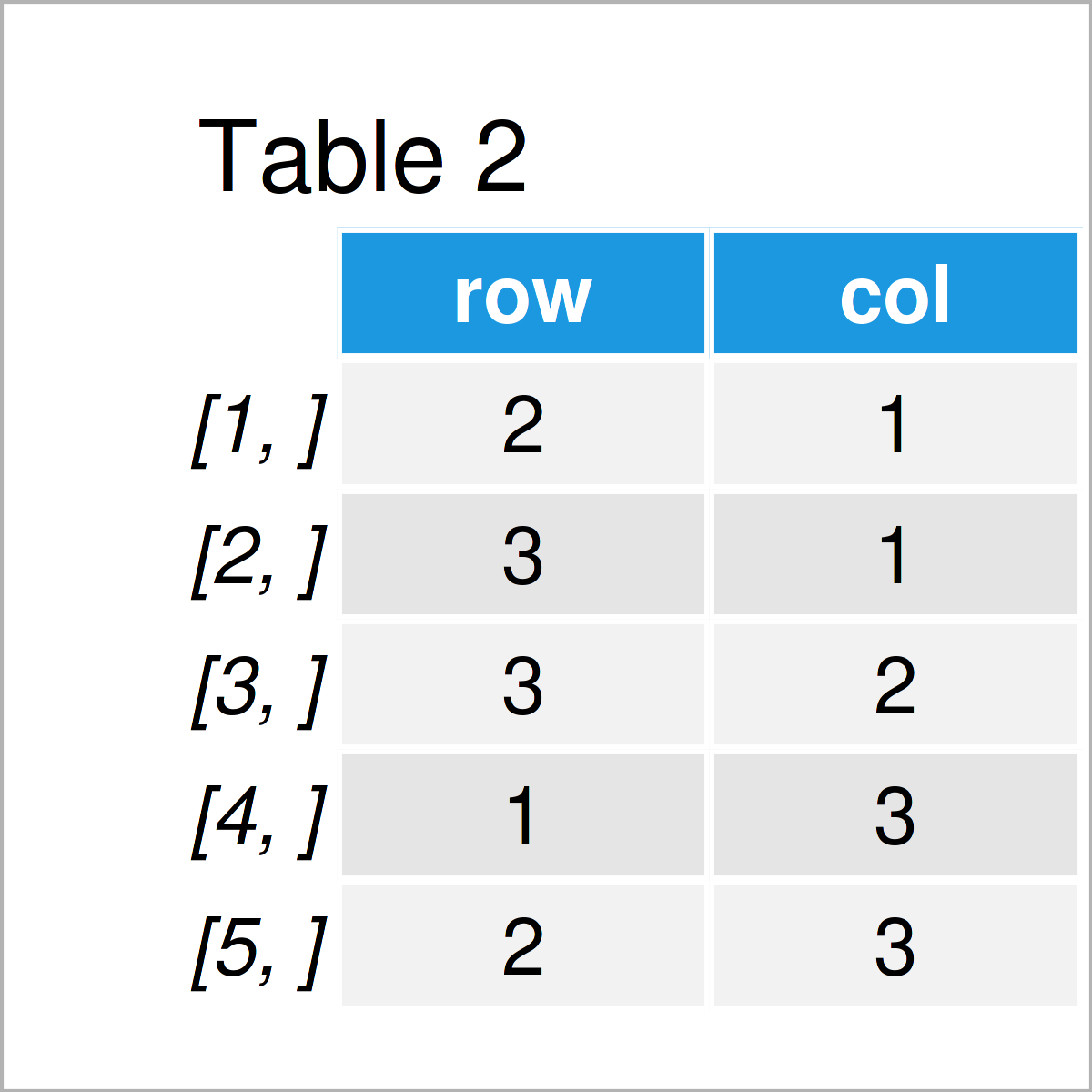# Find Index Positions of Non-Zero Values in Matrix in R (Example)

This article explains how to get the indices of non-zero values in a matrix in the R programming language.

The article will contain the following information:

Let’s dive into it:

## Example Data

The first step is to define some data that we can use in the examples later on:

```my_mat <- matrix(c(0, 1, 2, 0, 0, 2, 1, 2, 0),    # Create example matrix
ncol = 3)
my_mat                                            # Print example matrix```As you can see based on Table 1, our example data is a matrix consisting of three rows and three numerical columns.

Some of the values in our matrix are equal to zero, others are unequal to zero.

## Example: Identify Indices of Non-Zero Values in Matrix Using which() Function

The following syntax explains how to find the indices of all non-zero values in a matrix object.

For this task, we can apply the which. Within the which function, we have to set the arr.ind argument to be equal to TRUE:

```mat_nonzero <- which(my_mat != 0, arr.ind = T)    # Identify non-zero values
mat_nonzero                                       # Print matrix of non-zero locations```By running the previous R syntax, we have managed to construct Table 2, i.e. a matrix showing the row and column indices of non-zero numbers.

For instance, there is a non-zero value in the second row in the first column.

## Video, Further Resources & Summary

Have a look at the following video tutorial on my YouTube channel. I explain the R programming syntax of this tutorial in the video:

Please accept YouTube cookies to play this video. By accepting you will be accessing content from YouTube, a service provided by an external third party.If you accept this notice, your choice will be saved and the page will refresh.

Furthermore, you may want to have a look at the related tutorials on this website.